SNAP Library 6.0, Developer Reference  2020-12-09 16:24:20 SNAP, a general purpose, high performance system for analysis and manipulation of large networks
TNodeNet< TNodeData > Class Template Reference

Node Network (directed graph, TNGraph with data on nodes only). More...

`#include <network.h>`

Collaboration diagram for TNodeNet< TNodeData >: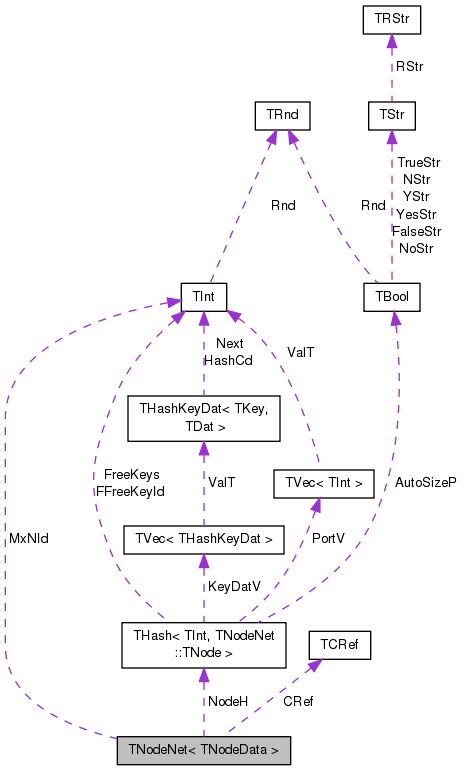[legend]

## Classes

class  TEdgeI
Edge iterator. Only forward iteration (operator++) is supported. More...

class  TNode

class  TNodeFunctor

class  TNodeI
Node iterator. Only forward iteration (operator++) is supported. More...

## Public Types

typedef TNodeData TNodeDat

typedef TNodeNet< TNodeData > TNet

typedef TPt< TNetPNet

## Public Member Functions

TNodeNet ()

TNodeNet (const int &Nodes, const int &Edges)
Constructor that reserves enough memory for a network of Nodes nodes and Edges edges. More...

TNodeNet (const TNodeNet &NodeNet)

TNodeNet (TSIn &SIn)
Constructor that loads the network from a (binary) stream SIn. More...

virtual ~TNodeNet ()

virtual void Save (TSOut &SOut) const
Saves the network to a (binary) stream SOut. More...

bool HasFlag (const TGraphFlag &Flag) const
Allows for run-time checking the type of the network (see the TGraphFlag for flags). More...

TNodeNetoperator= (const TNodeNet &NodeNet)

int GetNodes () const
Returns the number of nodes in the network. More...

Adds a node of ID NId to the network. More...

Adds a node of ID NId to the network, noop if the node already exists. More...

int AddNode (int NId, const TNodeData &NodeDat)
Adds a node of ID NId and node data NodeDat to the network. More...

Adds a node NodeI and its node data to the network. More...

virtual void DelNode (const int &NId)
Deletes node of ID NId from the network. More...

void DelNode (const TNode &NodeI)
Deletes node of ID NodeI.GetId() from the network. More...

bool IsNode (const int &NId) const
Tests whether ID NId is a node. More...

TNodeI BegNI () const
Returns an iterator referring to the first node in the network. More...

TNodeI EndNI () const
Returns an iterator referring to the past-the-end node in the network. More...

TNodeI GetNI (const int &NId) const
Returns an iterator referring to the node of ID NId in the network. More...

const TNodeGetNode (const int &NId) const
Returns node element for the node of ID NId in the network. More...

void SetNDat (const int &NId, const TNodeData &NodeDat)
Sets node data for the node of ID NId in the network. More...

TNodeData & GetNDat (const int &NId)
Returns node data for the node of ID NId in the network. More...

const TNodeData & GetNDat (const int &NId) const
Returns node data for the node of ID NId in the network. More...

int GetMxNId () const
Returns an ID that is larger than any node ID in the network. More...

int GetEdges () const
Returns the number of edges in the network. More...

int AddEdge (const int &SrcNId, const int &DstNId)
Adds an edge from node SrcNId to node DstNId to the network. More...

Adds an edge from EdgeI.GetSrcNId() to EdgeI.GetDstNId() to the network. More...

void DelEdge (const int &SrcNId, const int &DstNId, const bool &IsDir=true)
Deletes an edge from node IDs SrcNId to DstNId from the network. More...

bool IsEdge (const int &SrcNId, const int &DstNId, const bool &IsDir=true) const
Tests whether an edge from node IDs SrcNId to DstNId exists in the network. More...

TEdgeI BegEI () const
Returns an iterator referring to the first edge in the network. More...

TEdgeI EndEI () const
Returns an iterator referring to the past-the-end edge in the network. More...

TEdgeI GetEI (const int &EId) const
Not supported/implemented! More...

TEdgeI GetEI (const int &SrcNId, const int &DstNId) const
Returns an iterator referring to edge (SrcNId, DstNId) in the network. More...

int GetRndNId (TRnd &Rnd=TInt::Rnd)
Returns an ID of a random node in the network. More...

TNodeI GetRndNI (TRnd &Rnd=TInt::Rnd)
Returns an interator referring to a random node in the network. More...

void GetNIdV (TIntV &NIdV) const
Gets a vector IDs of all nodes in the network. More...

bool Empty () const
Tests whether the network is empty (has zero nodes). More...

void Clr (const bool &DoDel=true, const bool &ResetDat=true)
Deletes all nodes and edges from the network. More...

void Reserve (const int &Nodes, const int &Edges)
Reserves memory for a network of Nodes nodes and Edges edges. More...

void SortNIdById (const bool &Asc=true)
Sorts nodes by node IDs. More...

void SortNIdByDat (const bool &Asc=true)
Sorts nodes by node data. More...

Defragments the network. More...

bool IsOk (const bool &ThrowExcept=true) const
Checks the network data structure for internal consistency. More...

## Static Public Member Functions

static PNet New ()
Static constructor that returns a pointer to the network. Call: TPt <TNodeNet<TNodeData> > Net = TNodeNet<TNodeData>::New(). More...

Static constructor that loads the network from a stream SIn and returns a pointer to it. More...

Static constructor that loads the network from shared memory. More...

## Protected Member Functions

TNodeGetNode (const int &NId)

## Protected Attributes

TCRef CRef

TInt MxNId

THash< TInt, TNodeNodeH

## Friends

class TPt< TNodeNet< TNodeData > >

## Detailed Description

### template<class TNodeData> class TNodeNet< TNodeData >

Node Network (directed graph, TNGraph with data on nodes only).

Definition at line 17 of file network.h.

## Member Typedef Documentation

template<class TNodeData>
 typedef TPt TNodeNet< TNodeData >::PNet

Definition at line 21 of file network.h.

template<class TNodeData>
 typedef TNodeNet TNodeNet< TNodeData >::TNet

Definition at line 20 of file network.h.

template<class TNodeData>
 typedef TNodeData TNodeNet< TNodeData >::TNodeDat

Definition at line 19 of file network.h.

## Constructor & Destructor Documentation

template<class TNodeData>
 TNodeNet< TNodeData >::TNodeNet ( )
inline

Definition at line 160 of file network.h.

Referenced by TNodeNet< TSecTm >::Load(), TNodeNet< TSecTm >::LoadShM(), and TNodeNet< TSecTm >::New().

160 : CRef(), MxNId(0), NodeH() { }
THash< TInt, TNode > NodeH
Definition: network.h:144
TCRef CRef
Definition: network.h:142
TInt MxNId
Definition: network.h:143

Here is the caller graph for this function: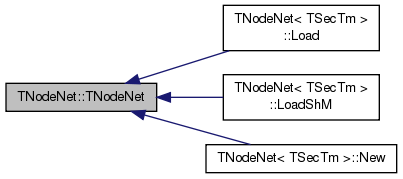template<class TNodeData>
 TNodeNet< TNodeData >::TNodeNet ( const int & Nodes, const int & Edges )
inlineexplicit

Constructor that reserves enough memory for a network of Nodes nodes and Edges edges.

Definition at line 162 of file network.h.

162 : MxNId(0) { Reserve(Nodes, Edges); }
TInt MxNId
Definition: network.h:143
void Reserve(const int &Nodes, const int &Edges)
Reserves memory for a network of Nodes nodes and Edges edges.
Definition: network.h:278
template<class TNodeData>
 TNodeNet< TNodeData >::TNodeNet ( const TNodeNet< TNodeData > & NodeNet )
inline

Definition at line 163 of file network.h.

163 : MxNId(NodeNet.MxNId), NodeH(NodeNet.NodeH) { }
THash< TInt, TNode > NodeH
Definition: network.h:144
TInt MxNId
Definition: network.h:143
template<class TNodeData>
 TNodeNet< TNodeData >::TNodeNet ( TSIn & SIn )
inline

Constructor that loads the network from a (binary) stream SIn.

Definition at line 165 of file network.h.

165 : MxNId(SIn), NodeH(SIn) { }
THash< TInt, TNode > NodeH
Definition: network.h:144
TInt MxNId
Definition: network.h:143
template<class TNodeData>
 virtual TNodeNet< TNodeData >::~TNodeNet ( )
inlinevirtual

Definition at line 166 of file network.h.

166 { }

## Member Function Documentation

template<class TNodeData >
 int TNodeNet< TNodeData >::AddEdge ( const int & SrcNId, const int & DstNId )

Adds an edge from node SrcNId to node DstNId to the network.

If the edge already exists return -2. If the edge was successfully added return -1. Normally the function should return an ID of the edge added but since edges in TNodeNet have no IDs we return -1. Function aborts if SrcNId or DstNId are not nodes in the network.

Definition at line 381 of file network.h.

References TStr::Fmt(), and IAssertR.

381  {
382  IAssertR(IsNode(SrcNId) && IsNode(DstNId), TStr::Fmt("%d or %d not a node.", SrcNId, DstNId).CStr());
383  if (IsEdge(SrcNId, DstNId)) { return -2; }
386  return -1; // no edge id
387 }
#define IAssertR(Cond, Reason)
Definition: bd.h:265
TNode & GetNode(const int &NId)
Definition: network.h:139
TIntV InNIdV
Definition: network.h:27
TSizeTy AddSorted(const TVal &Val, const bool &Asc=true, const TSizeTy &_MxVals=-1)
Adds element Val to a sorted vector.
Definition: ds.h:1117
bool IsNode(const int &NId) const
Tests whether ID NId is a node.
Definition: network.h:217
bool IsEdge(const int &SrcNId, const int &DstNId, const bool &IsDir=true) const
Tests whether an edge from node IDs SrcNId to DstNId exists in the network.
Definition: network.h:401
static TStr Fmt(const char *FmtStr,...)
Definition: dt.cpp:1599
TIntV OutNIdV
Definition: network.h:27

Here is the call graph for this function:Here is the caller graph for this function: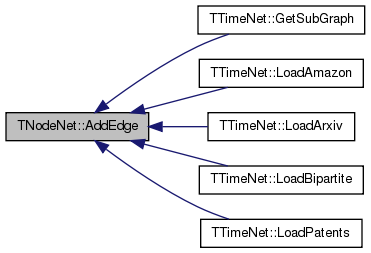template<class TNodeData>
 int TNodeNet< TNodeData >::AddEdge ( const TEdgeI & EdgeI )
inline

Adds an edge from EdgeI.GetSrcNId() to EdgeI.GetDstNId() to the network.

Definition at line 247 of file network.h.

247 { return AddEdge(EdgeI.GetSrcNId(), EdgeI.GetDstNId()); }
int AddEdge(const int &SrcNId, const int &DstNId)
Adds an edge from node SrcNId to node DstNId to the network.
Definition: network.h:381

Here is the caller graph for this function: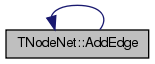template<class TNodeData >
 int TNodeNet< TNodeData >::AddNode ( int NId = `-1` )

Adds a node of ID NId to the network.

Returns the ID of the node being added. If NId is -1, node ID is automatically assigned. Aborts, if a node with ID NId already exists.

Definition at line 311 of file network.h.

References TStr::Fmt(), IAssertR, and TMath::Mx().

311  {
312  if (NId == -1) {
313  NId = MxNId; MxNId++;
314  } else {
315  IAssertR(!IsNode(NId), TStr::Fmt("NodeId %d already exists", NId));
316  MxNId = TMath::Mx(NId+1, MxNId());
317  }
319  return NId;
320 }
#define IAssertR(Cond, Reason)
Definition: bd.h:265
static const T & Mx(const T &LVal, const T &RVal)
Definition: xmath.h:32
THash< TInt, TNode > NodeH
Definition: network.h:144
bool IsNode(const int &NId) const
Tests whether ID NId is a node.
Definition: network.h:217
TInt MxNId
Definition: network.h:143
static TStr Fmt(const char *FmtStr,...)
Definition: dt.cpp:1599
Definition: hash.h:238

Here is the call graph for this function: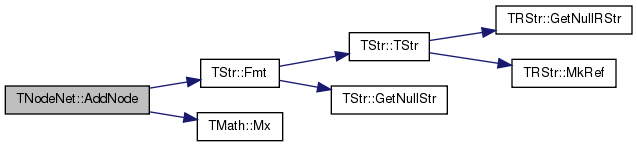Here is the caller graph for this function: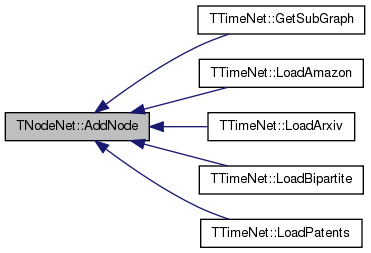template<class TNodeData>
 int TNodeNet< TNodeData >::AddNode ( int NId, const TNodeData & NodeDat )

Adds a node of ID NId and node data NodeDat to the network.

Returns the ID of the node being added. If NId is -1, node ID is automatically assigned. Aborts, if a node with ID NId already exists.

Definition at line 335 of file network.h.

References TStr::Fmt(), IAssertR, and TMath::Mx().

335  {
336  if (NId == -1) {
337  NId = MxNId; MxNId++;
338  } else {
339  IAssertR(!IsNode(NId), TStr::Fmt("NodeId %d already exists", NId));
340  MxNId = TMath::Mx(NId+1, MxNId());
341  }
343  return NId;
344 }
#define IAssertR(Cond, Reason)
Definition: bd.h:265
static const T & Mx(const T &LVal, const T &RVal)
Definition: xmath.h:32
THash< TInt, TNode > NodeH
Definition: network.h:144
bool IsNode(const int &NId) const
Tests whether ID NId is a node.
Definition: network.h:217
TInt MxNId
Definition: network.h:143
static TStr Fmt(const char *FmtStr,...)
Definition: dt.cpp:1599
Definition: hash.h:238

Here is the call graph for this function:template<class TNodeData>
 int TNodeNet< TNodeData >::AddNode ( const TNodeI & NodeI )
inline

Adds a node NodeI and its node data to the network.

Definition at line 209 of file network.h.

209 { return AddNode(NodeI.GetId(), NodeI.GetDat()); }
Adds a node of ID NId to the network.
Definition: network.h:311

Here is the caller graph for this function: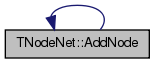template<class TNodeData >
 int TNodeNet< TNodeData >::AddNodeUnchecked ( int NId = `-1` )

Adds a node of ID NId to the network, noop if the node already exists.

Returns -1, if the node NId already exists. Otherwise, it returns the ID of the node being added. If NId is -1, node ID is automatically assigned.

Definition at line 323 of file network.h.

References TMath::Mx().

323  {
324  if (NId == -1) {
325  NId = MxNId; MxNId++;
326  } else {
327  if (IsNode(NId)) { return -1;}
328  MxNId = TMath::Mx(NId+1, MxNId());
329  }
331  return NId;
332 }
static const T & Mx(const T &LVal, const T &RVal)
Definition: xmath.h:32
THash< TInt, TNode > NodeH
Definition: network.h:144
bool IsNode(const int &NId) const
Tests whether ID NId is a node.
Definition: network.h:217
TInt MxNId
Definition: network.h:143
Definition: hash.h:238

Here is the call graph for this function: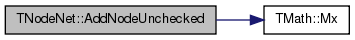template<class TNodeData>
 TEdgeI TNodeNet< TNodeData >::BegEI ( ) const
inline

Returns an iterator referring to the first edge in the network.

Definition at line 257 of file network.h.

257 { TNodeI NI=BegNI(); while(NI<EndNI() && NI.GetOutDeg()==0) NI++; return TEdgeI(NI, EndNI()); }
TNodeI EndNI() const
Returns an iterator referring to the past-the-end node in the network.
Definition: network.h:221
TNodeI BegNI() const
Returns an iterator referring to the first node in the network.
Definition: network.h:219
int GetOutDeg() const
Returns out-degree of the current node.
Definition: network.h:80
template<class TNodeData>
 TNodeI TNodeNet< TNodeData >::BegNI ( ) const
inline

Returns an iterator referring to the first node in the network.

Definition at line 219 of file network.h.

219 { return TNodeI(NodeH.BegI(), this); }
TIter BegI() const
Definition: hash.h:213
THash< TInt, TNode > NodeH
Definition: network.h:144

Here is the caller graph for this function: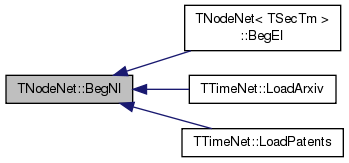template<class TNodeData>
 void TNodeNet< TNodeData >::Clr ( const bool & DoDel = `true`, const bool & ResetDat = `true` )
inline

Deletes all nodes and edges from the network.

Definition at line 275 of file network.h.

275  {
276  MxNId = 0; NodeH.Clr(DoDel, -1, ResetDat); }
THash< TInt, TNode > NodeH
Definition: network.h:144
TInt MxNId
Definition: network.h:143
void Clr(const bool &DoDel=true, const int &NoDelLim=-1, const bool &ResetDat=true)
Definition: hash.h:361
template<class TNodeData >
 void TNodeNet< TNodeData >::Defrag ( const bool & OnlyNodeLinks = `false` )

Defragments the network.

After performing many node and edge insertions and deletions to a network, the network data structure will be fragmented in memory. This function compacts down the network data structure and frees unneeded memory.

Definition at line 423 of file network.h.

423  {
424  for (int n = NodeH.FFirstKeyId(); NodeH.FNextKeyId(n); ) {
425  TNode& Node = NodeH[n];
426  Node.InNIdV.Pack(); Node.OutNIdV.Pack();
427  }
428  if (! OnlyNodeLinks && ! NodeH.IsKeyIdEqKeyN()) {
429  NodeH.Defrag(); }
430 }
bool IsKeyIdEqKeyN() const
Definition: hash.h:233
THash< TInt, TNode > NodeH
Definition: network.h:144
void Defrag()
Definition: hash.h:555
bool FNextKeyId(int &KeyId) const
Definition: hash.h:478
int FFirstKeyId() const
Definition: hash.h:278
void Pack()
Definition: hash.h:289

Here is the call graph for this function: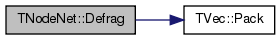Here is the caller graph for this function: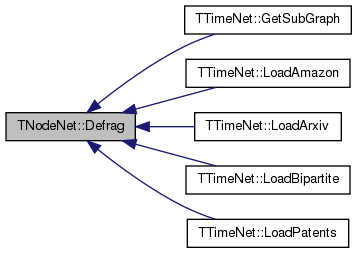template<class TNodeData >
 void TNodeNet< TNodeData >::DelEdge ( const int & SrcNId, const int & DstNId, const bool & IsDir = `true` )

Deletes an edge from node IDs SrcNId to DstNId from the network.

If the edge (SrcNId, DstNId) does not exist in the network function still completes. But the function aborts if SrcNId or DstNId are not nodes in the network.

Definition at line 390 of file network.h.

References TStr::Fmt(), and IAssertR.

390  {
391  IAssertR(IsNode(SrcNId) && IsNode(DstNId), TStr::Fmt("%d or %d not a node.", SrcNId, DstNId).CStr());
392  GetNode(SrcNId).OutNIdV.DelIfIn(DstNId);
393  GetNode(DstNId).InNIdV.DelIfIn(SrcNId);
394  if (! IsDir) {
395  GetNode(DstNId).OutNIdV.DelIfIn(SrcNId);
396  GetNode(SrcNId).InNIdV.DelIfIn(DstNId);
397  }
398 }
bool DelIfIn(const TVal &Val)
Removes the first occurrence of element Val.
Definition: ds.h:1212
#define IAssertR(Cond, Reason)
Definition: bd.h:265
TNode & GetNode(const int &NId)
Definition: network.h:139
TIntV InNIdV
Definition: network.h:27
bool IsNode(const int &NId) const
Tests whether ID NId is a node.
Definition: network.h:217
static TStr Fmt(const char *FmtStr,...)
Definition: dt.cpp:1599
TIntV OutNIdV
Definition: network.h:27

Here is the call graph for this function:template<class TNodeData >
 void TNodeNet< TNodeData >::DelNode ( const int & NId )
virtual

Deletes node of ID NId from the network.

If the node of ID NId does not exist the function aborts.

Definition at line 347 of file network.h.

347  {
348  { TNode& Node = GetNode(NId);
349  for (int e = 0; e < Node.GetOutDeg(); e++) {
350  const int nbr = Node.GetOutNId(e);
351  if (nbr == NId) { continue; }
352  TNode& N = GetNode(nbr);
353  int n = N.InNIdV.SearchBin(NId);
354  if (n!= -1) { N.InNIdV.Del(n); }
355  }
356  for (int e = 0; e < Node.GetInDeg(); e++) {
357  const int nbr = Node.GetInNId(e);
358  if (nbr == NId) { continue; }
359  TNode& N = GetNode(nbr);
360  int n = N.OutNIdV.SearchBin(NId);
361  if (n!= -1) { N.OutNIdV.Del(n); }
362  } }
363  NodeH.DelKey(NId);
364 }
TNode & GetNode(const int &NId)
Definition: network.h:139
THash< TInt, TNode > NodeH
Definition: network.h:144
void DelKey(const TKey &Key)
Definition: hash.h:404

Here is the call graph for this function: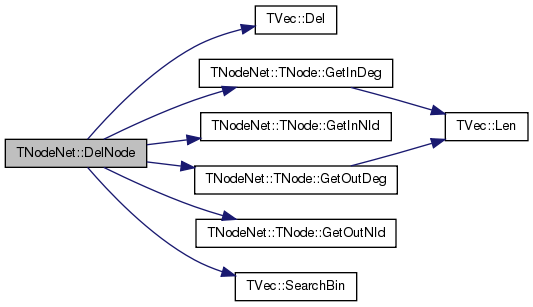Here is the caller graph for this function:template<class TNodeData>
 void TNodeNet< TNodeData >::DelNode ( const TNode & NodeI )
inline

Deletes node of ID NodeI.GetId() from the network.

Definition at line 215 of file network.h.

Referenced by TNodeNet< TSecTm >::DelNode().

215 { DelNode(NodeI.GetId()); }
virtual void DelNode(const int &NId)
Deletes node of ID NId from the network.
Definition: network.h:347

Here is the caller graph for this function: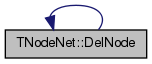template<class TNodeData>
 bool TNodeNet< TNodeData >::Empty ( ) const
inline

Tests whether the network is empty (has zero nodes).

Definition at line 273 of file network.h.

273 { return GetNodes()==0; }
int GetNodes() const
Returns the number of nodes in the network.
Definition: network.h:189
template<class TNodeData>
 TEdgeI TNodeNet< TNodeData >::EndEI ( ) const
inline

Returns an iterator referring to the past-the-end edge in the network.

Definition at line 259 of file network.h.

259 { return TEdgeI(EndNI(), EndNI()); }
TNodeI EndNI() const
Returns an iterator referring to the past-the-end node in the network.
Definition: network.h:221
template<class TNodeData>
 TNodeI TNodeNet< TNodeData >::EndNI ( ) const
inline

Returns an iterator referring to the past-the-end node in the network.

Definition at line 221 of file network.h.

221 { return TNodeI(NodeH.EndI(), this); }
THash< TInt, TNode > NodeH
Definition: network.h:144
TIter EndI() const
Definition: hash.h:218

Here is the caller graph for this function: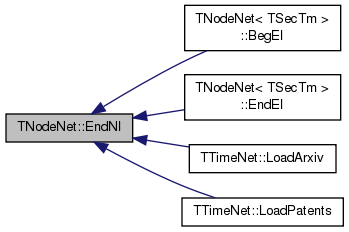template<class TNodeData >
 int TNodeNet< TNodeData >::GetEdges ( ) const

Returns the number of edges in the network.

Definition at line 373 of file network.h.

373  {
374  int edges=0;
375  for (int N=NodeH.FFirstKeyId(); NodeH.FNextKeyId(N);) {
376  edges+=NodeH[N].GetOutDeg(); }
377  return edges;
378 }
THash< TInt, TNode > NodeH
Definition: network.h:144
bool FNextKeyId(int &KeyId) const
Definition: hash.h:478
int FFirstKeyId() const
Definition: hash.h:278

Here is the caller graph for this function: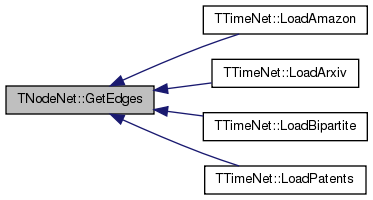template<class TNodeData>
 TEdgeI TNodeNet< TNodeData >::GetEI ( const int & EId ) const

Not supported/implemented!

template<class TNodeData >
 TNodeNet< TNodeData >::TEdgeI TNodeNet< TNodeData >::GetEI ( const int & SrcNId, const int & DstNId ) const

Returns an iterator referring to edge (SrcNId, DstNId) in the network.

Definition at line 415 of file network.h.

References TNodeNet< TNodeData >::TNodeI::NodeHI.

415  {
416  const TNodeI SrcNI = GetNI(SrcNId);
417  const int NodeN = SrcNI.NodeHI.GetDat().OutNIdV.SearchBin(DstNId);
418  if (NodeN == -1) { return EndEI(); }
419  return TEdgeI(SrcNI, EndNI(), NodeN);
420 }
TNodeI EndNI() const
Returns an iterator referring to the past-the-end node in the network.
Definition: network.h:221
TNodeI GetNI(const int &NId) const
Returns an iterator referring to the node of ID NId in the network.
Definition: network.h:223
TEdgeI EndEI() const
Returns an iterator referring to the past-the-end edge in the network.
Definition: network.h:259
template<class TNodeData>
 int TNodeNet< TNodeData >::GetMxNId ( ) const
inline

Returns an ID that is larger than any node ID in the network.

Definition at line 233 of file network.h.

233 { return MxNId; }
TInt MxNId
Definition: network.h:143
template<class TNodeData>
 TNodeData& TNodeNet< TNodeData >::GetNDat ( const int & NId )
inline

Returns node data for the node of ID NId in the network.

Definition at line 229 of file network.h.

229 { return NodeH.GetDat(NId).NodeDat; }
THash< TInt, TNode > NodeH
Definition: network.h:144
const TDat & GetDat(const TKey &Key) const
Definition: hash.h:262
TNodeData NodeDat
Definition: network.h:26

Here is the caller graph for this function: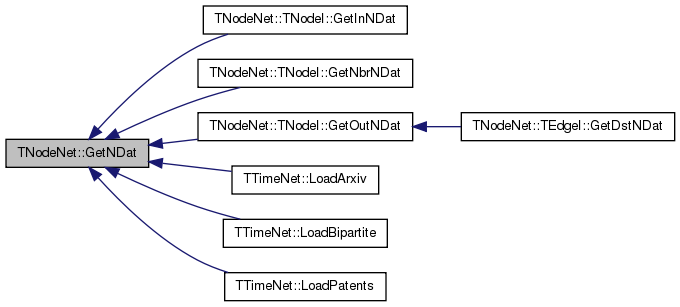template<class TNodeData>
 const TNodeData& TNodeNet< TNodeData >::GetNDat ( const int & NId ) const
inline

Returns node data for the node of ID NId in the network.

Definition at line 231 of file network.h.

231 { return NodeH.GetDat(NId).NodeDat; }
THash< TInt, TNode > NodeH
Definition: network.h:144
const TDat & GetDat(const TKey &Key) const
Definition: hash.h:262
TNodeData NodeDat
Definition: network.h:26
template<class TNodeData>
 TNodeI TNodeNet< TNodeData >::GetNI ( const int & NId ) const
inline

Returns an iterator referring to the node of ID NId in the network.

Definition at line 223 of file network.h.

Referenced by TNodeNet< TSecTm >::GetRndNI().

223 { return TNodeI(NodeH.GetI(NId), this); }
THash< TInt, TNode > NodeH
Definition: network.h:144
TIter GetI(const TKey &Key) const
Definition: hash.h:220

Here is the caller graph for this function: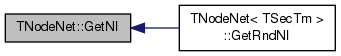template<class TNodeData >
 void TNodeNet< TNodeData >::GetNIdV ( TIntV & NIdV ) const

Gets a vector IDs of all nodes in the network.

Definition at line 408 of file network.h.

References TVec< TVal, TSizeTy >::Add(), and TVec< TVal, TSizeTy >::Reserve().

408  {
409  NIdV.Reserve(GetNodes(), 0);
410  for (int N=NodeH.FFirstKeyId(); NodeH.FNextKeyId(N); ) {
412 }
THash< TInt, TNode > NodeH
Definition: network.h:144
int GetNodes() const
Returns the number of nodes in the network.
Definition: network.h:189
bool FNextKeyId(int &KeyId) const
Definition: hash.h:478
int FFirstKeyId() const
Definition: hash.h:278
void Reserve(const TSizeTy &_MxVals)
Reserves enough memory for the vector to store _MxVals elements.
Definition: ds.h:543
Adds a new element at the end of the vector, after its current last element.
Definition: ds.h:602
const TKey & GetKey(const int &KeyId) const
Definition: hash.h:252

Here is the call graph for this function:template<class TNodeData>
 TNode& TNodeNet< TNodeData >::GetNode ( const int & NId )
inlineprotected

Definition at line 139 of file network.h.

139 { return NodeH.GetDat(NId); }
THash< TInt, TNode > NodeH
Definition: network.h:144
const TDat & GetDat(const TKey &Key) const
Definition: hash.h:262
template<class TNodeData>
 const TNode& TNodeNet< TNodeData >::GetNode ( const int & NId ) const
inline

Returns node element for the node of ID NId in the network.

Definition at line 225 of file network.h.

225 { return NodeH.GetDat(NId); }
THash< TInt, TNode > NodeH
Definition: network.h:144
const TDat & GetDat(const TKey &Key) const
Definition: hash.h:262
template<class TNodeData>
 int TNodeNet< TNodeData >::GetNodes ( ) const
inline

Returns the number of nodes in the network.

Definition at line 189 of file network.h.

189 { return NodeH.Len(); }
THash< TInt, TNode > NodeH
Definition: network.h:144
int Len() const
Definition: hash.h:228

Here is the caller graph for this function: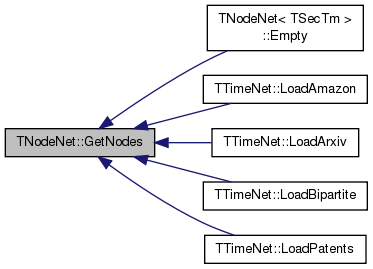template<class TNodeData>
 TNodeI TNodeNet< TNodeData >::GetRndNI ( TRnd & Rnd = `TInt::Rnd` )
inline

Returns an interator referring to a random node in the network.

Definition at line 268 of file network.h.

268 { return GetNI(GetRndNId(Rnd)); }
TNodeI GetNI(const int &NId) const
Returns an iterator referring to the node of ID NId in the network.
Definition: network.h:223
int GetRndNId(TRnd &Rnd=TInt::Rnd)
Returns an ID of a random node in the network.
Definition: network.h:266
template<class TNodeData>
 int TNodeNet< TNodeData >::GetRndNId ( TRnd & Rnd = `TInt::Rnd` )
inline

Returns an ID of a random node in the network.

Definition at line 266 of file network.h.

Referenced by TNodeNet< TSecTm >::GetRndNI().

266 { return NodeH.GetKey(NodeH.GetRndKeyId(Rnd, 0.8)); }
THash< TInt, TNode > NodeH
Definition: network.h:144
int GetRndKeyId(TRnd &Rnd) const
Get an index of a random element. If the hash table has many deleted keys, this may take a long time...
Definition: hash.h:444
const TKey & GetKey(const int &KeyId) const
Definition: hash.h:252

Here is the caller graph for this function: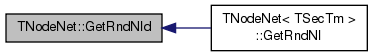template<class TNodeData >
 bool TNodeNet< TNodeData >::HasFlag ( const TGraphFlag & Flag ) const

Allows for run-time checking the type of the network (see the TGraphFlag for flags).

Definition at line 306 of file network.h.

References HasGraphFlag.

306  {
307  return HasGraphFlag(typename TNet, Flag);
308 }
#define HasGraphFlag(TGraph, Flag)
For quick testing of the properties of the graph/network object (see TGraphFlag). ...
Definition: gbase.h:41
TNodeNet< TNodeData > TNet
Definition: network.h:20
template<class TNodeData >
 bool TNodeNet< TNodeData >::IsEdge ( const int & SrcNId, const int & DstNId, const bool & IsDir = `true` ) const

Tests whether an edge from node IDs SrcNId to DstNId exists in the network.

Definition at line 401 of file network.h.

401  {
402  if (! IsNode(SrcNId) || ! IsNode(DstNId)) { return false; }
403  if (IsDir) { return GetNode(SrcNId).IsOutNId(DstNId); }
404  else { return GetNode(SrcNId).IsOutNId(DstNId) || GetNode(DstNId).IsOutNId(SrcNId); }
405 }
bool IsOutNId(const int &NId) const
Definition: network.h:45
TNode & GetNode(const int &NId)
Definition: network.h:139
bool IsNode(const int &NId) const
Tests whether ID NId is a node.
Definition: network.h:217

Here is the caller graph for this function: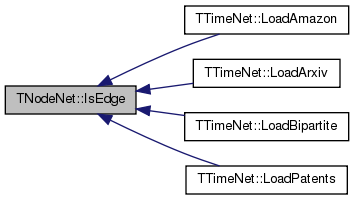template<class TNodeData>
 bool TNodeNet< TNodeData >::IsNode ( const int & NId ) const
inline

Tests whether ID NId is a node.

Definition at line 217 of file network.h.

217 { return NodeH.IsKey(NId); }
THash< TInt, TNode > NodeH
Definition: network.h:144
bool IsKey(const TKey &Key) const
Definition: hash.h:258

Here is the caller graph for this function: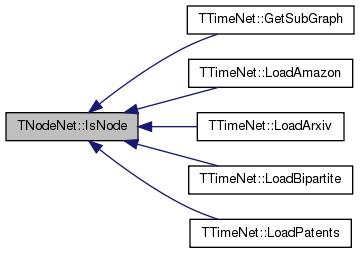template<class TNodeData >
 bool TNodeNet< TNodeData >::IsOk ( const bool & ThrowExcept = `true` ) const

Checks the network data structure for internal consistency.

For each node in the network check that its neighbors are also nodes in the network.

Definition at line 433 of file network.h.

433  {
434  bool RetVal = true;
435  for (int N = NodeH.FFirstKeyId(); NodeH.FNextKeyId(N); ) {
436  const TNode& Node = NodeH[N];
437  if (! Node.OutNIdV.IsSorted()) {
438  const TStr Msg = TStr::Fmt("Out-neighbor list of node %d is not sorted.", Node.GetId());
439  if (ThrowExcept) { EAssertR(false, Msg); } else { ErrNotify(Msg.CStr()); } RetVal=false;
440  }
441  if (! Node.InNIdV.IsSorted()) {
442  const TStr Msg = TStr::Fmt("In-neighbor list of node %d is not sorted.", Node.GetId());
443  if (ThrowExcept) { EAssertR(false, Msg); } else { ErrNotify(Msg.CStr()); } RetVal=false;
444  }
445  // check out-edges
446  int prevNId = -1;
447  for (int e = 0; e < Node.GetOutDeg(); e++) {
448  if (! IsNode(Node.GetOutNId(e))) {
449  const TStr Msg = TStr::Fmt("Out-edge %d --> %d: node %d does not exist.",
450  Node.GetId(), Node.GetOutNId(e), Node.GetOutNId(e));
451  if (ThrowExcept) { EAssertR(false, Msg); } else { ErrNotify(Msg.CStr()); } RetVal=false;
452  }
453  if (e > 0 && prevNId == Node.GetOutNId(e)) {
454  const TStr Msg = TStr::Fmt("Node %d has duplidate out-edge %d --> %d.",
455  Node.GetId(), Node.GetId(), Node.GetOutNId(e));
456  if (ThrowExcept) { EAssertR(false, Msg); } else { ErrNotify(Msg.CStr()); } RetVal=false;
457  }
458  prevNId = Node.GetOutNId(e);
459  }
460  // check in-edges
461  prevNId = -1;
462  for (int e = 0; e < Node.GetInDeg(); e++) {
463  if (! IsNode(Node.GetInNId(e))) {
464  const TStr Msg = TStr::Fmt("In-edge %d <-- %d: node %d does not exist.",
465  Node.GetId(), Node.GetInNId(e), Node.GetInNId(e));
466  if (ThrowExcept) { EAssertR(false, Msg); } else { ErrNotify(Msg.CStr()); } RetVal=false;
467  }
468  if (e > 0 && prevNId == Node.GetInNId(e)) {
469  const TStr Msg = TStr::Fmt("Node %d has duplidate in-edge %d <-- %d.",
470  Node.GetId(), Node.GetId(), Node.GetInNId(e));
471  if (ThrowExcept) { EAssertR(false, Msg); } else { ErrNotify(Msg.CStr()); } RetVal=false;
472  }
473  prevNId = Node.GetInNId(e);
474  }
475  }
476  return RetVal;
477 }
THash< TInt, TNode > NodeH
Definition: network.h:144
void ErrNotify(const char *NotifyCStr)
Definition: bd.h:74
bool IsNode(const int &NId) const
Tests whether ID NId is a node.
Definition: network.h:217
bool FNextKeyId(int &KeyId) const
Definition: hash.h:478
int FFirstKeyId() const
Definition: hash.h:278
Definition: dt.h:412
static TStr Fmt(const char *FmtStr,...)
Definition: dt.cpp:1599
#define EAssertR(Cond, MsgStr)
Definition: bd.h:283
char * CStr()
Definition: dt.h:479

Here is the call graph for this function: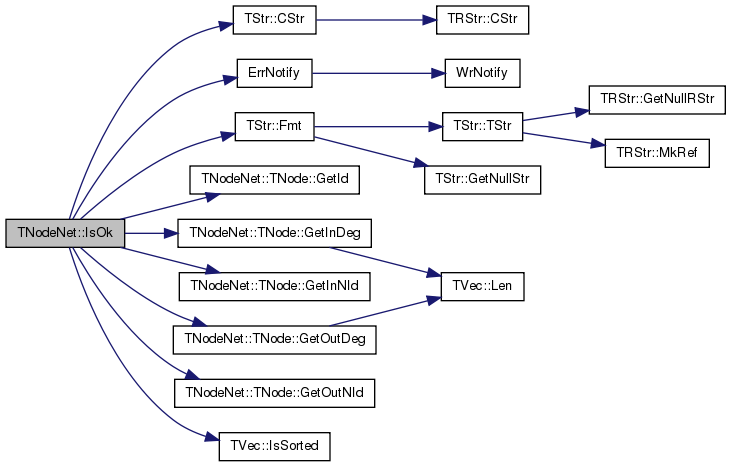template<class TNodeData>
 static PNet TNodeNet< TNodeData >::Load ( TSIn & SIn )
inlinestatic

Static constructor that loads the network from a stream SIn and returns a pointer to it.

Definition at line 172 of file network.h.

172 { return PNet(new TNodeNet(SIn)); }
TNodeNet()
Definition: network.h:160
TPt< TNet > PNet
Definition: network.h:21
template<class TNodeData>
 void TNodeNet< TNodeData >::LoadNetworkShM ( TShMIn & ShMIn )
inlineprivate

Definition at line 153 of file network.h.

153  {
154  MxNId = TInt(ShMIn);
155  TNodeFunctor f;
157  }
THash< TInt, TNode > NodeH
Definition: network.h:144
Load THash from shared memory file. Copying/Deleting Keys is illegal.
Definition: hash.h:157
TInt MxNId
Definition: network.h:143
Definition: dt.h:1137

Here is the caller graph for this function: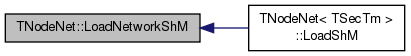template<class TNodeData>
 static PNet TNodeNet< TNodeData >::LoadShM ( TShMIn & ShMIn )
inlinestatic

Static constructor that loads the network from shared memory.

The object is read-only. It does not support operations that edit the edge vectors of nodes or perform illegal operations on the NodeH, EdgeH (deletion or swapping keys).

Definition at line 178 of file network.h.

178  {
179  TNodeNet* Network = new TNodeNet();
181  return PNet(Network);
182  }
Definition: network.h:153
TNodeNet()
Definition: network.h:160
TPt< TNet > PNet
Definition: network.h:21
Node Network (directed graph, TNGraph with data on nodes only).
Definition: network.h:17
template<class TNodeData>
 static PNet TNodeNet< TNodeData >::New ( )
inlinestatic

Static constructor that returns a pointer to the network. Call: TPt <TNodeNet<TNodeData> > Net = TNodeNet<TNodeData>::New().

Definition at line 170 of file network.h.

170 { return PNet(new TNodeNet()); }
TNodeNet()
Definition: network.h:160
TPt< TNet > PNet
Definition: network.h:21
template<class TNodeData>
 TNodeNet& TNodeNet< TNodeData >::operator= ( const TNodeNet< TNodeData > & NodeNet )
inline

Definition at line 185 of file network.h.

185  {
186  if (this!=&NodeNet) { NodeH=NodeNet.NodeH; MxNId=NodeNet.MxNId; } return *this; }
THash< TInt, TNode > NodeH
Definition: network.h:144
TInt MxNId
Definition: network.h:143
template<class TNodeData>
 void TNodeNet< TNodeData >::Reserve ( const int & Nodes, const int & Edges )
inline

Reserves memory for a network of Nodes nodes and Edges edges.

Definition at line 278 of file network.h.

278 { if (Nodes>0) { NodeH.Gen(Nodes/2); } }
THash< TInt, TNode > NodeH
Definition: network.h:144
void Gen(const int &ExpectVals)
Definition: hash.h:222

Here is the caller graph for this function: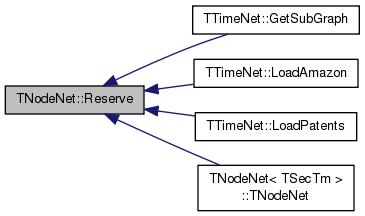template<class TNodeData>
 virtual void TNodeNet< TNodeData >::Save ( TSOut & SOut ) const
inlinevirtual

Saves the network to a (binary) stream SOut.

Reimplemented in TTimeNet.

Definition at line 168 of file network.h.

168 { MxNId.Save(SOut); NodeH.Save(SOut); }
void Save(TSOut &SOut) const
Definition: dt.h:1153
void Save(TSOut &SOut) const
Definition: hash.h:183
THash< TInt, TNode > NodeH
Definition: network.h:144
TInt MxNId
Definition: network.h:143
template<class TNodeData>
 void TNodeNet< TNodeData >::SetNDat ( const int & NId, const TNodeData & NodeDat )

Sets node data for the node of ID NId in the network.

Definition at line 367 of file network.h.

References TStr::Fmt(), and IAssertR.

367  {
368  IAssertR(IsNode(NId), TStr::Fmt("NodeId %d does not exist.", NId).CStr());
369  NodeH.GetDat(NId).NodeDat = NodeDat;
370 }
#define IAssertR(Cond, Reason)
Definition: bd.h:265
THash< TInt, TNode > NodeH
Definition: network.h:144
const TDat & GetDat(const TKey &Key) const
Definition: hash.h:262
TNodeData NodeDat
Definition: network.h:26
bool IsNode(const int &NId) const
Tests whether ID NId is a node.
Definition: network.h:217
static TStr Fmt(const char *FmtStr,...)
Definition: dt.cpp:1599

Here is the call graph for this function:template<class TNodeData>
 void TNodeNet< TNodeData >::SortNIdByDat ( const bool & Asc = `true` )
inline

Sorts nodes by node data.

Definition at line 282 of file network.h.

282 { NodeH.SortByDat(Asc); }
THash< TInt, TNode > NodeH
Definition: network.h:144
void SortByDat(const bool &Asc=true)
Definition: hash.h:292
template<class TNodeData>
 void TNodeNet< TNodeData >::SortNIdById ( const bool & Asc = `true` )
inline

Sorts nodes by node IDs.

Definition at line 280 of file network.h.

280 { NodeH.SortByKey(Asc); }
THash< TInt, TNode > NodeH
Definition: network.h:144
void SortByKey(const bool &Asc=true)
Definition: hash.h:291

## Friends And Related Function Documentation

template<class TNodeData>
 friend class TPt< TNodeNet< TNodeData > >
friend

Definition at line 296 of file network.h.

## Member Data Documentation

template<class TNodeData>
 TCRef TNodeNet< TNodeData >::CRef
protected

Definition at line 142 of file network.h.

template<class TNodeData>
 TInt TNodeNet< TNodeData >::MxNId
protected

Definition at line 143 of file network.h.

Referenced by TNodeNet< TSecTm >::GetMxNId(), and TNodeNet< TSecTm >::operator=().

template<class TNodeData>
 THash TNodeNet< TNodeData >::NodeH
protected

Definition at line 144 of file network.h.

Referenced by TNodeNet< TSecTm >::GetNode(), and TNodeNet< TSecTm >::operator=().

The documentation for this class was generated from the following file: